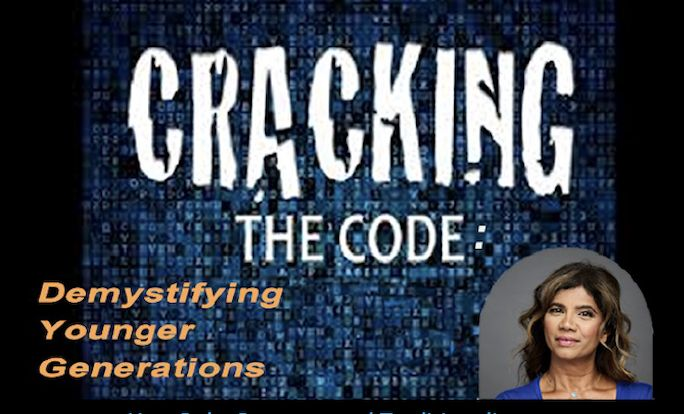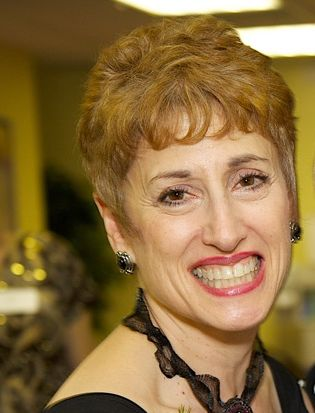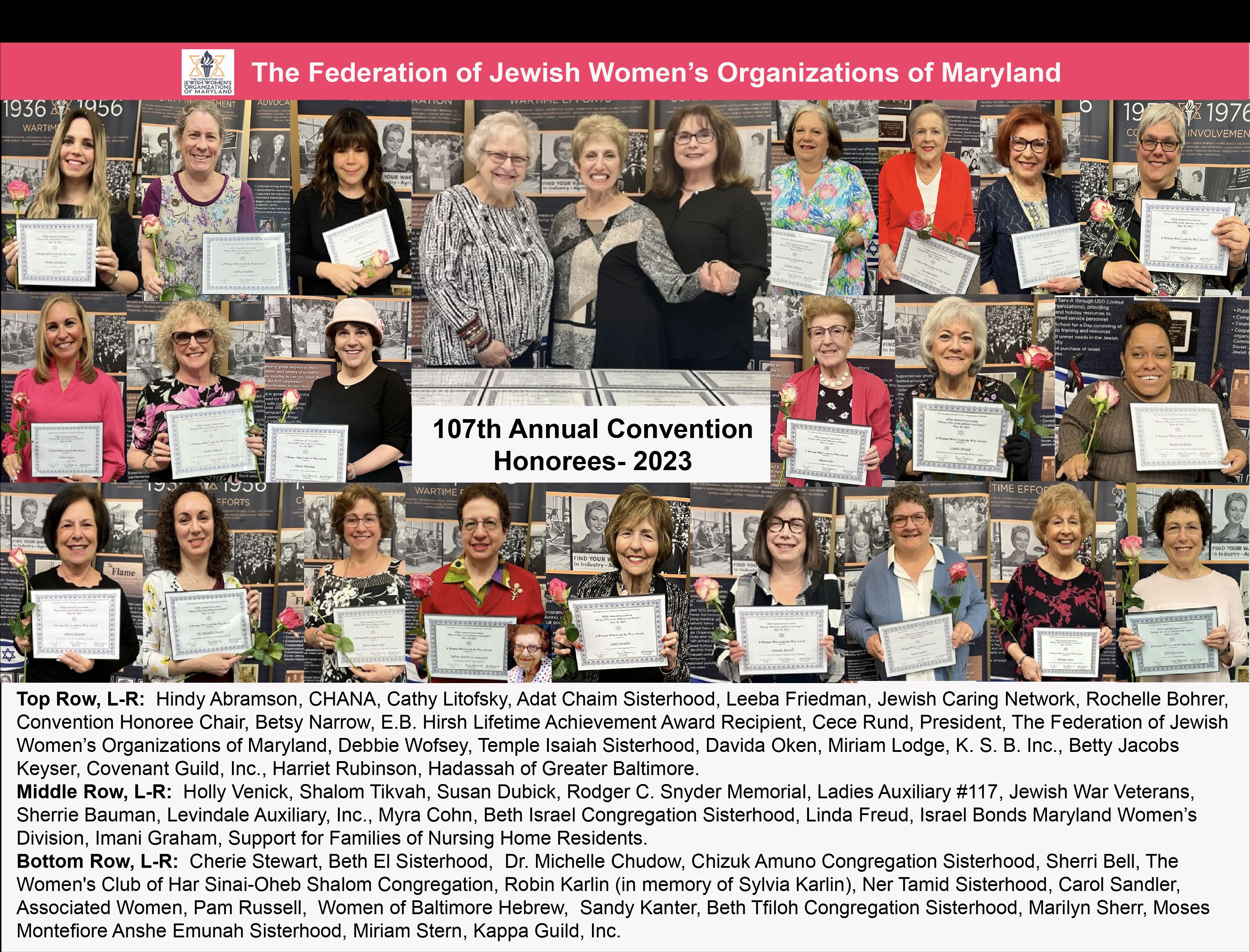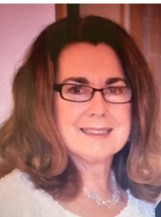The Federation is the oldest and only Jewish Women's
Federation in the Country - Since 1916

*ALL MEMBERS OF OUR CONSTITUENT ORGANIZATIONS
ARE MEMBERS OF FEDERATION

<<<<<<<<<<<<<<<<<<<<<<<<<<<<<<<<<<<<<<<<<<<<<<<<<<<<<<<<<<<Visit and Like us on Facebook to Stay Informed!

<<<<<<<<<<<<<<<<<<<<<<<<<<<<<<<<<<<<<<<<<<<<<<<<<<<<<<<<<<<The Federation of Jewish Women's Organizations of Maryland shares our shock and horror at the vicious Hammas attacks on Israel; it's land,
it's people and it's right to be. We support the IDF and Israel's right to defend itself against terrorists actions and attacks. We pray for our people. We pray for the IDF soldiers to be kept safe. We pray for the Israeli government to stand brave and to make the right decisions and to
stand as an example for the world.

<<<<<<<<<<<<<<<<<<<<<<<<<<<<<<<<<<<<<<<<<<<<<<<<<<<<<<<<<<<

MID-WINTER CONFERENCE
Thursday, January 25, 2024
9:30 a.m.
Held on ZoomGuest Speaker: Rekha C. Gibbons,
Chief Optimism Officer at Raare Solutions, NYC

How Baby Boomers and Tradionalists can understand and communicate with the younger generation

<<<<<<<<<<<<<<<<<<<<<<<<<<<<<<<<<<<<<<<<<<<<<<<<<<<<<<<<<<<

Thank you for making this year's
107th Annual Convention
such a success!!!

MAZEL TOV to
Betsy Narrow, E.B. Hirsh Lifetime Achievement Award RecipientVIEW OUR HONOREES RECEIVING THE "WOMAN WHO LEADS THE WAY" AWARD

"A Woman Who Leads the Way"
Award Honorees
Visit Award page to see our
2023 Award HonoreesMazel Tov Honoree Form

<<<<<<<<<<<<<<<<<<<<<<<<<<<<<<<<<<<<<<<<<<<<<<<<<<<<<<<<<<<

What is Serv-A?

<<<<<<<<<<<<<<<<<<<<<<<<<<<<<<<<<<<<<<<<<<<<<<<<<<<<<<<<<<<

President, CeCe Rund<<<<<<<<<<<<<<<<<<<<<<<<<<<<<<<<<<<<<<<<<<<<<<<<<<<<<<<<<<<

Officers for 2023-2024
President - CeCe Rund
First Vice President - Shulamit Finkelstein
Vice President - Julie Bernstein
Vice President - Edie Meyers
Corresponding Secretaries - Rochelle Bohrer, Ferne Rogow
Recording Secretary - Eve Kresin Steinberg
Treasurer - Ann Fishkin
Auditor - Rosalind Asch
Community Representative - Marcia Bornfirend, Past President

<<<<<<<<<<<<<<<<<<<<<<<<<<<<<<<<<<<<<<<<<<<<<<<<<<<<<<<<<<<

 The Federation of Jewish Women's Organizations of Maryland is the Oldest Jewish Women's Federation in the Country Founded 1916Calendar To see the full calendar and Jewish holidays, CLICK HERE.